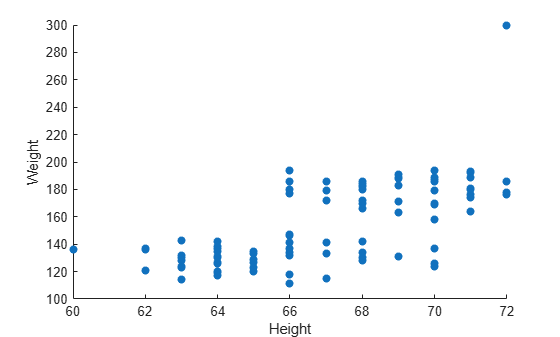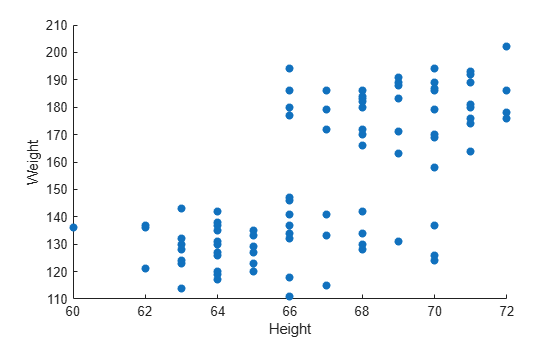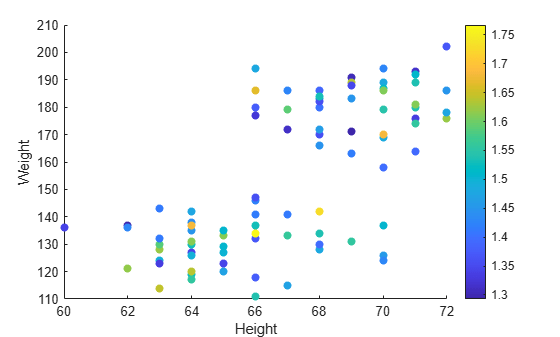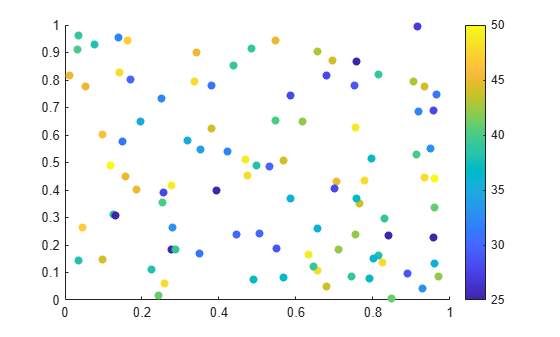## Plots That Support Tables

Many plotting functions can plot data directly from a table. You pass the table as the first argument to the function followed by the variables you want to plot. You can specify either a table or a timetable, and in many cases, you can plot multiple data sets together in the same axes.

The following examples use the `scatter` and `bubblechart` functions to demonstrate the overall approach for plotting data from a table. To learn if a specific plotting function supports tables, refer to the documentation for that function.

### Create Scatter Plot from a Table

Read `patients.xls` as a table and plot the `Diastolic` variable versus the `Systolic` variable. By default, the axis labels are automatically set to the variable names.

```tbl = readtable("patients.xls"); figure scatter(tbl,"Systolic","Diastolic")```Plot both blood pressure measurements against the `Weight` variable and specify filled markers. Then add a legend. By default, the plot uses different colors for the systolic and diastolic measurements, and the legend labels display the variable names.

Because the y-coordinates come from two different table variables, it is not clear what the y-axis label should be, so the label remains blank.

```scatter(tbl,"Weight",["Systolic","Diastolic"],"filled") legend```### Update Plot by Modifying the Table

When you pass a table to a plotting function, a copy of the table is stored in the `SourceTable` property of the plot object. If you change the contents of the table stored in that property, the plot is automatically updated to show the changes.

Read `patients.xls` as a table and plot the `Weight` variable versus the `Height` variable. Return the `Scatter` object as `s`, so you can access its properties later.

```tbl = readtable("patients.xls"); figure s = scatter(tbl,"Height","Weight","filled");```To change a value in the table, use dot notation to reference the table from the `SourceTable` property of the `Scatter` object. In this case, find the maximum value of the `Weight` variable and change it to `300`. The plot is automatically updated.

Note: Making changes to a table in your workspace has no effect on the plot.

```[~,idx] = max(s.SourceTable.Weight); s.SourceTable.Weight(idx) = 300;```### Customize Marker Appearance with Table Variables

For some plots, you can vary the color, size, and transparency of the markers in your plot according to certain variables in your table. Some functions have arguments for specifying those aspects. For example, create a bubble chart of the patients data set. Plot the `Weight` variable versus the `Height` variable. Use the fourth argument to vary bubble sizes according to the `Diastolic` variable. Use the fifth argument to vary the color according to the `Age` variable.

Add a colorbar and resize the bubbles so that they range between `5` and `20` points.

```tbl = readtable("patients.xls"); figure bubblechart(tbl,"Height","Weight","Diastolic","Age") % Add a colorbar and resize the bubbles colorbar bubblesize([5 20])```When you use the `scatter` function, you can use name-value arguments or set properties on the `Scatter` object to control different aspects of the markers. For example, create a scatter plot with filled markers, and set the `ColorVariable` property by specifying a name-value argument. Then add a colorbar.

```figure s = scatter(tbl,"Height","Weight","filled","ColorVariable","Age"); colorbar```Next, vary the transparency of the markers according to the `Systolic` variable. To do this, you must also set the `MarkerFaceAlpha` property to `'flat'`.

```s.AlphaVariable = "Systolic"; s.MarkerFaceAlpha = "flat";```### Plot Table Coordinates with Scalar Marker Size

To specify a scalar marker size when creating a scatter plot from a table, use the `SizeData` name-value argument.

For example, read `patients.xls` as a table and plot the `Weight` variable versus the `Height` variable. Use the `SizeData` name-value argument to specify 200-point markers.

```tbl = readtable("patients.xls"); figure scatter(tbl,"Weight","Height","SizeData",200)```### Combine Table and Vector Data

Many plots that support tables allow you to specify some aspects of your plot using a table variable and other aspects using vectors or matrices. For example, you can create a scatter plot using coordinates from a table and customize the colors of the markers by setting the `CData` property to a vector, an RGB triplet, or a matrix of RGB triplets.

Create a scatter plot using data from a table. Read `patients.xls` as a table, and plot the `Weight` versus the `Height` variables.

```tbl = readtable("patients.xls"); figure s = scatter(tbl,"Height","Weight","filled");```Next, change the colors of the plotted points using a vector. When you combine data from different sources like this, the size of each vector, matrix, or table variable must be compatible with the plot you are creating. In this case, create a vector called `bpratio` by dividing the systolic values by the diastolic values from the table. Because `bpratio` is derived from the same table as the `Height` and `Weight` variables, it has the same number of elements as those variables, and so it is compatible with this plot.

Color each point according to the blood pressure ratio by setting the `CData` property to `bpratio`. Then add a colorbar.

```% Vary the color by blood pressure ratio bpratio = tbl.Systolic./tbl.Diastolic; s.CData = bpratio; % Add a colorbar colorbar```You can also plot vectors or matrices, and modify the plot using table variables. After you create the plot, set the `SourceTable` property, and then set the table-related properties that you want. Table-related properties typically have the word `Variable` in their names. For example, plot two vectors of 100 random numbers.

```x = rand(100,1); y = rand(100,1); figure s = scatter(x,y,"filled");```Change the marker colors so that they vary according to the values in a table variable. Read `patients.xls` as the table `tbl`. Set the `SourceTable` property and vary the marker colors according to the `Age` variable in the table. Because the table has 100 rows, and the plot has 100 points, the `Age` variable is compatible with the plot. Then, add a colorbar to the plot.

```% Set source table and vary color by age s.SourceTable = tbl; s.ColorVariable = "Age"; % Add a colorbar colorbar```Note: Standalone visualizations such as `heatmap` do not support combinations of table and vector data.